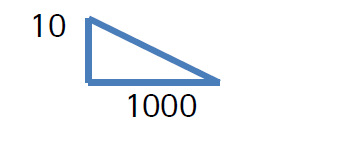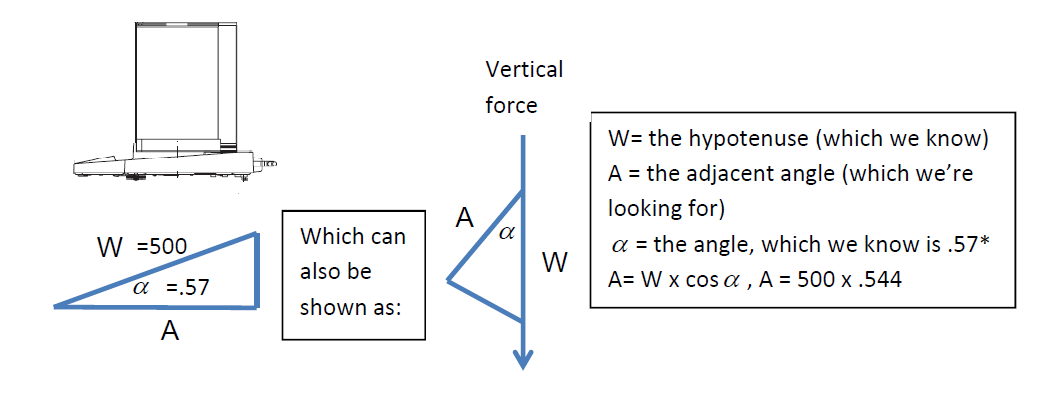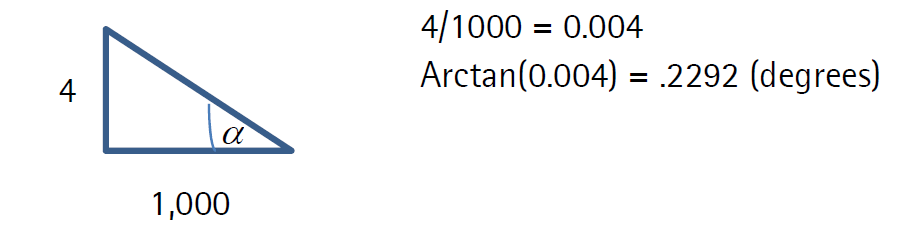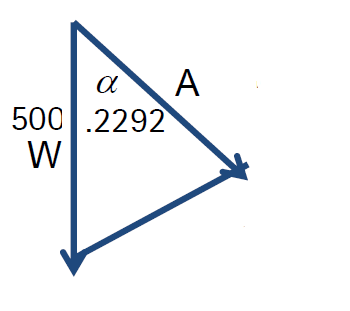# Slope’s Effect on Accurate WeighingSlope’s Effect on Accurate Weighing

July 15, 2014Customers often have concerns that their floor isn’t level and questions as to what affect that will have on their weight readings. The scale is designed with the assumption that the load will be parallel to the direction of gravity and perpendicular to the weighing platform; meaning, there is only a vertical force. An uneven balance or scale causes the introduction of a horizontal force that affects the weight reading. The general rule of thumb is that if it’s an industrial application, such as a floor scale, as long as all of the feet are touching the ground the results will be within tolerance. For laboratories, however, and customers who want more than a general “rule of thumb” response, there is actually a procedure for determining the exact effect the slope will have on the measurement. By looking at the formulas below, the next time a customer calls and asks about floor specifications, you can provide them with an exact mathematic response.
For a quick trigonometry refresher let’s go over a couple of definitions. The first will be the arctangent, or inverse tangent. Arctangent is used to calculate the angles of a right triangle when the two sides – adjacent and opposite – are known. This will be used to help calculate the degree of incline (the angle). The second is the cosine. The cosine is used when the adjacent and hypotenuse angles are known, or in our case, the hypotenuse and the angle are known, which will determine the adjacent side. It may sound confusing, but we will walk through an example that should help clarify.
Say you have a weight of 500kg. Placed on a level scale, the result will (or should be without technical failures) 500kg. Now, say the scale is on a floor with a slope of 10mm per 1000mm.The slope will be 10/1000 or 1/100, which equals 0.01. Now, since we know the two sides we can use the arctangent to determine the angle. So take 0.01 and multiply by arctan (or inverse tan if your calculator doesn’t have an arctangent function). The rounded answer comes to 0.57*. This is the slope of your angle in degrees.
So what do we do now that we know the angle? In the below example the “W” stands for the actual weight being applied, or 500kg. This will also be the “length” of that side, the hypotenuse. The “A” represents the adjacent side, which is the actual weight that is going to be displayed given the angle. We also know that alpha = 0.57*. So, as we discussed above, if we know the hypotenuse and the angle we can determine the adjacent side. So, 500 x (cos).57 = 500 x .99995, = 499.975. So side “A” = 499.975 which is also the weight reading that will be displayed, 25g off from the actual weight being applied (drawings are not to scale, for reference only).Depending on the industrial application this may or may not be a significant error. Let’s take a look at an example of when this out of level is applied in laboratory applications.

Let’s say we are weighing expensive API (Active Pharmaceutical Ingredients) in 500mg increments on a micro balance (0.001mg). The balance is leveled at calibration, but now a 20kg box of product has been placed in the middle of the bench. The bench now goes out of level and without a re-adjustment of the balance the weighing pan will now be out of level as well. The new slope is 4mm per 1000mm. First we need to find the angle and since we know the opposite and adjacent sides we will use the arctangent function:Now we have our degree of incline, which we will use for our next equation:We know the side of the hypotenuse, W, is 500mg. We will use this as the “length” of that side. We know that the angle (alpha) is .2292. We also know that when we know the hypotenuse side and the angle we will use the cosine function. So, A = W x Cos a. Cos a = .999991 so the equation becomes A=500 x .999991 = A = 499.9955, or .0045mg off of the actual weight being applied.
Depending on the price of the API being weighed, this could be a significant error over the course of time, resulting in a lot of money being lost for a relatively simple fix. Many lab balances today are equipped with auto out-of-level indication and some even perform the level adjustment automatically to avoid playing with the feet for 20 minutes to get the bubble perfectly centered.
Evan Foisy is marketing manager at Sartorious Intec USA Inc.

For related articles, equipment reviews, and news, visit our Weighing Systems & Scales Equipment Zone